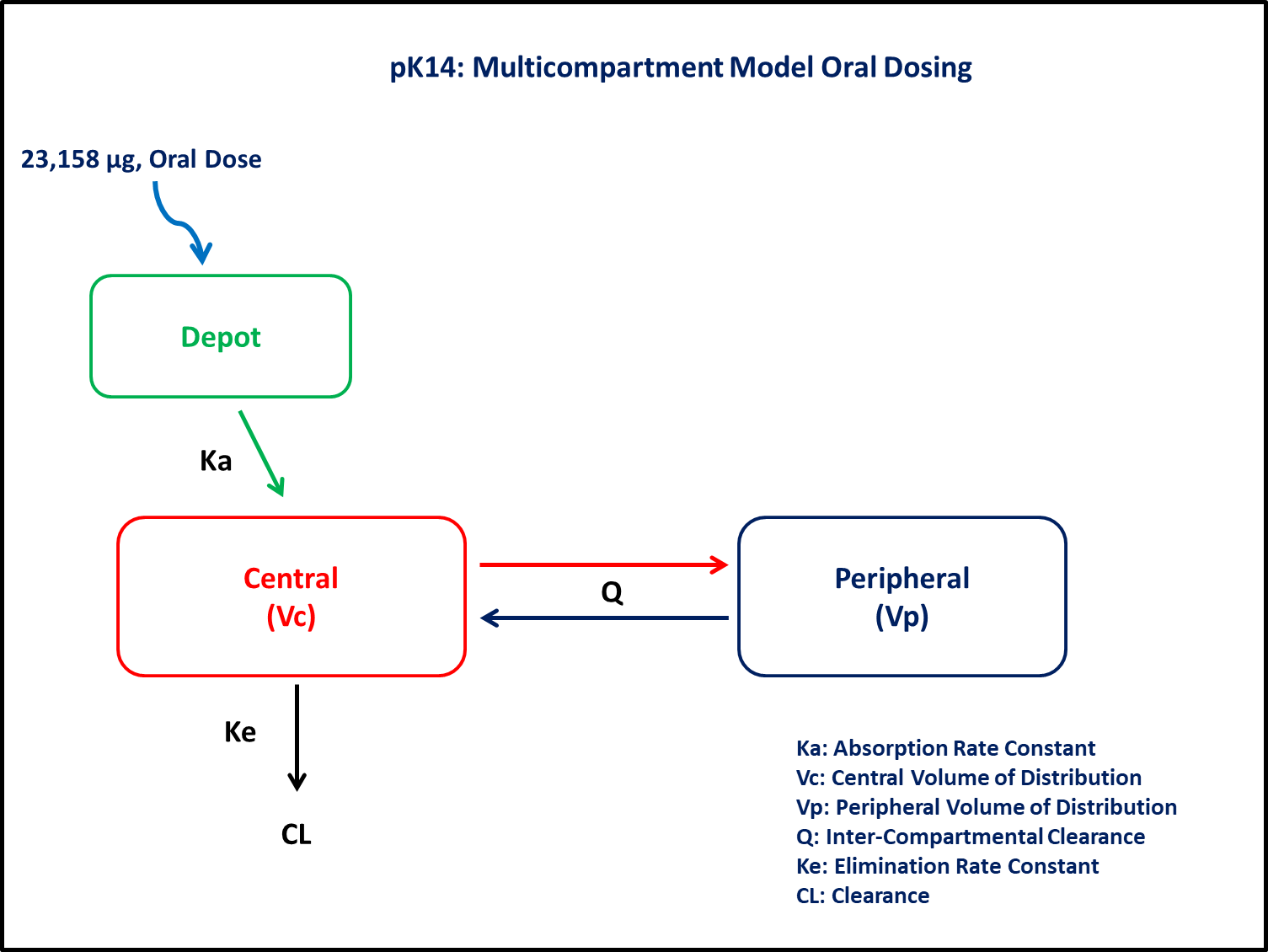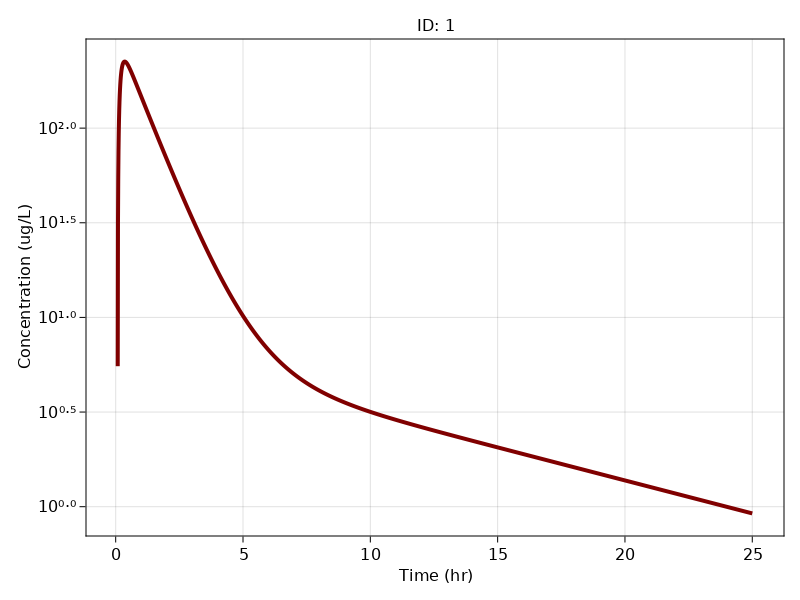# Exercise PK14 - Multi-compartment oral data

### Background

• Structural model - Two compartment linear elimination and first order absorption with lagtime

• Route of administration - Oral

• Dosage regimens - 23,158 μg single dose

• Subject - 1### Learning Outcome

In this model, we will simulate an oral dose to understand the disposition of drug following lag time in absorption

### Objectives

In this tutorial, you will learn how to build two compartment model with lagtime in oral absorption and to simulate the model for a single subject.

### Libraries

Call the "necessary" libraries to get start.

using Random
using Pumas
using PumasUtilities
using CairoMakie


### Model

In this two compartment model, we administer dose to Depot and Central compartment.

pk_14           = @model begin
desc        = "Two Compartment Model"
timeu       = u"hr"
end

@param begin
"Absorption Rate Constant (hr⁻¹)"
tvka        ∈ RealDomain(lower=0)
"Volume of Central Compartment (L)"
tvvc        ∈ RealDomain(lower=0)
"Volume of Peripheral Compartment (L)"
tvvp        ∈ RealDomain(lower=0)
"Clearance (L/hr)"
tvcl        ∈ RealDomain(lower=0)
"Intercompartmental Clearance (L/hr)"
tvq         ∈ RealDomain(lower=0)
"Lagtime (hr)"
tvlag       ∈ RealDomain(lower=0)
Ω           ∈ PDiagDomain(4)
"Proportional RUV"
σ²_prop     ∈ RealDomain(lower=0)
end

@random begin
η           ~ MvNormal(Ω)
end

@pre begin
Ka          = tvka * exp(η)
Vc          = tvvc * exp(η)
Vp          = tvvp * exp(η)
CL          = tvcl * exp(η)
Q           = tvq
end

@dosecontrol begin
lags        = (Depot = tvlag,)
end

@dynamics Depots1Central1Periph1

@derived begin
cp          = @. Central/Vc
"""
Observed Concentrations (ug/L)
"""
dv          ~ @. Normal(cp, sqrt(cp^2*σ²_prop))
end
end

PumasModel
Parameters: tvka, tvvc, tvvp, tvcl, tvq, tvlag, Ω, σ²_prop
Random effects: η
Covariates:
Dynamical variables: Depot, Central, Peripheral
Derived: cp, dv
Observed: cp, dv


### Parameters

Parameters provided for simulation. tv represents the typical value for parameters.

• $Ka$ - Absorption rate constant (hr⁻¹)

• $Vc$ - Volume of central compartment (L)

• $Vp$ - Volume of peripheral Compartmental (L)

• $CL$ - Clearance (L/hr)

• $Q$ - Intercompartmental clearance (L/hr)

• $lag$ - Absorption lagtime (hr)

param = ( tvka    = 10,
tvvc    = 82.95,
tvcl    = 54.87,
tvq     = 10.55,
tvlag   = 0.078,
tvvp    = 107.9,
Ω       = Diagonal([0.0,0.0,0.0,0.0]),
σ²_prop = 0.0125)

(tvka = 10, tvvc = 82.95, tvcl = 54.87, tvq = 10.55, tvlag = 0.078, tvvp =
107.9, Ω = [0.0 0.0 0.0 0.0; 0.0 0.0 0.0 0.0; 0.0 0.0 0.0 0.0; 0.0 0.0 0.0
0.0], σ²_prop = 0.0125)


### Dosage Regimen

Single Subject receives an oral dose of 23158 μg at time=0

ev1  = DosageRegimen(23158, time = 0, cmt = 1)
sub1 = Subject(id = 1, events = ev1)

Subject
ID: 1
Events: 1


### Simulation

Lets simulate plasma concentration after oral dosing.

Random.seed!(123)
sim_sub1 = simobs(pk_14, sub1, param, obstimes=0.08:0.01:25)


### Visualization

sim_plot(pk_14, [sim_sub1],
observations = :cp,
linewidth = 4,
color = :maroon,
axis = (xlabel = "Time (hr)",
ylabel="Concentration (ug/L)",
yscale = log10, xticks = 0:5:25))